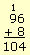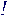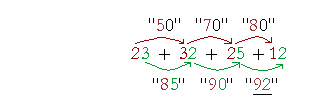S k i l l
i n
A R I T H M E T I C

Lesson 5

## Mental calculation

In these pages we emphasize problems that do not require pencil and paper, and certainly not a calculator. For as we point out in the Introduction, arithmetic is a spoken skill, based on knowing the addition and multiplication tables. Although we use the term mental calculation, we mean by speaking, whether mentally or aloud.

In this Lesson, we will answer the following:

The student should now have mastered the elementary addition of one-digit numbers, and should be practicing the multiplication table.

For, once you know that

 5 + 4 = 9, then you would also know: 50 + 40  = 90, 500 + 400  = 900, 5,000 + 4,000  = 9,000

These are not problems to write in a column.

Our first skill is based on the following properties of addition:

 1. A sum does not change if we decompose a term into two or more terms. 25 + 7 = 25 + 5 + 2 = 32. 2. A sum does not change no matter how we group the terms. 8 + 6 + 2 + 4 = (8 + 2) + (6 + 4) = 20.

One should look, then, for numbers that add up to 10.

Essential for mental calculation is knowing the multiples of 10 --

10,  20,  30,  40,  50,  and so on

-- and being able to count by 10's.  See Elementary Addition.

 1. How do we add mentally by composing a multiple of 10? 17 + 5 = 17 + 3 + 2 = 20 + 2 = 22. Decompose -- break up -- the smaller number and regroup with the larger to form the next multiple of 10. Then add what remains of the smaller number.
 Example 1. 38 + 4.
 Say, "38 plus 2 is 40, plus 2 is 42."
 Example 1. 59 + 7. " 59 plus 1 is 60, plus 6 is 66."
 Example 1. 96 + 8. " 96 plus 4 is 100, plus 4 is 104."

To add 96 + 8, then, an educated person does not have to take out a

pencil, write in a column and say,"6 plus 8 is 14 -- write 4, carry 1.  etc., etc., etc."

Nor does an educated person count on her fingers.  Rather, an educated person knows elementary addition, and therefore that 96 + 8 is not very different from 6 + 8.  It ends in 4.

Example 2.   Since

9 + 6 = 15,

then

 90 + 60 = 150 \$.90 + \$.60 = \$1.50 \$.90 + \$.64 = \$1.54

A 9 plus a 6 always gives a "15."  This brings us to the practical technique for adding several numbers --

7 + 8 + 3 + 9 + 4

 2. What does it mean to add by endings? It means to know the digit in which the sum will end.

For example, since

8 + 4 = 12,

you would know that if you add any number ending in 8 to any number ending in 4, the sum will always end in 2.

28 + 4 = 32

38 + 4 = 42

68 + 4 = 72

Similarly, since

6 + 5 = 11

then

26 + 5 = 31

46 + 5 = 51

76 + 5 = 81

They all end in 1.  These are not problems to write down.

Example 3.   Add from left to right.

 7 + 8 + 3 + 8 + 6 + 4 + 9

Say only the partial sum as you come to it.  Do not say,
"7 plus 8 is 15" -- say only "15."  Then look at 3 and say 18.  And so on.  Use your knowledge of the ending; even exaggerate saying it.

 7 + 8 + 3 + 8 + 6 + 4 + 9 Say: "15 18 26 32 36 45."

Complements

Two numbers are called complementary if their sum is 10.  And since the order of terms does not matter, we may take advantage of complements.

 Example 4.    Add 6 + 4 + 3 + 1 + 8 + 7 + 2. Solution. 6 + 4 + 3 + 1 + 8 + 7 + 2 = 30 + 1 = 31.

 3. How do we add mentally by place value? Add the numbers as you read them -- from left to right. Add from higher place to lower.

435 + 461 = 896

First add the hundreds, then the tens, then the ones.

We now see the fundamental principle of all mental calculation:

Calculate from left to right, just as you read.
The last number you say is the answerExample 5.  Counting by 10's.    30 + 24  =  54.Here are other examples:

20 + 16 = 36

40 + 38 = 78

40 + 62 = 102

40 + 82 = 122

90 + 73 = 163

Example 6.     43 + 25

First add the tens then the ones.  Say

"Sixty --

43 + 25

-- eight."

Or, you could say,

"43 plus 20 is 63, plus 5 is 68."Say, "150 plus 7 is 157."Say only, "50 plus 14 is 64."

The art of mental calculation is to say as little as possible.  The last number you say is the answer."170 plus 11 is 181."

Example 10.    23 + 32 + 25 + 12

First add all the tens, then add on the ones.  As you add each place, say the partial sum.  SayThe last number you say is the entire sum.

 Example 11. 34 + 25 + 32"50,  80,  89,  91."

Example 12.   653 + 224

First add the hundreds, then the tens, then the ones.  Again, say each partial sum:

653 + 224

Say,

"800,  870,  877."

Example 13.    Three tracks on a CD have the following times:

10:34

6:25

8:07

What is the total time?

10:34 means 10 minutes 34 seconds.  60 seconds = 1 minute.

(Therefore, 72 seconds = 1 minute 12 seconds.  1:12.)

"16 plus 8 is 24 minutes."

"24:59 plus 7 is 24: 66."

The total time is 25 minutes 6 seconds.

At this point, please "turn" the page and do some Problems.

or

Continue on to Section 2: